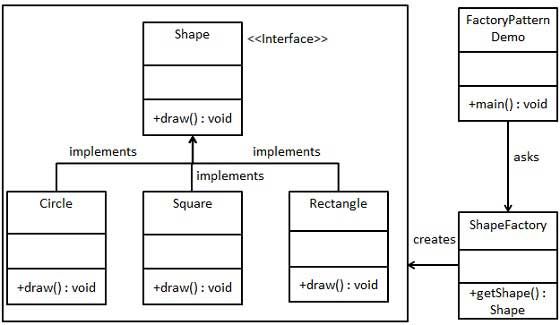# 工厂模式

## 实现

FactoryPatternDemo，我们的演示类使用 ShapeFactory 来获取 Shape 对象。它将向 ShapeFactory 传递信息（CIRCLE / RECTANGLE / SQUARE），以便获取它所需对象的类型。### 步骤 1

```public interface Shape {
void draw();
}```

### 步骤 2

```public class Rectangle implements Shape {

@Override
public void draw() {
System.out.println("Inside Rectangle::draw() method.");
}
}
```
```public class Square implements Shape {

@Override
public void draw() {
System.out.println("Inside Square::draw() method.");
}
}
```
```public class Circle implements Shape {

@Override
public void draw() {
System.out.println("Inside Circle::draw() method.");
}
}```

### 步骤 3

```public class ShapeFactory {

//使用 getShape 方法获取形状类型的对象
public Shape getShape(String shapeType){
if(shapeType == null){
return null;
}
if(shapeType.equalsIgnoreCase("CIRCLE")){
return new Circle();
} else if(shapeType.equalsIgnoreCase("RECTANGLE")){
return new Rectangle();
} else if(shapeType.equalsIgnoreCase("SQUARE")){
return new Square();
}
return null;
}
}```

### 步骤 4

```public class FactoryPatternDemo {

public static void main(String[] args) {
ShapeFactory shapeFactory = new ShapeFactory();

//获取 Circle 的对象，并调用它的 draw 方法
Shape shape1 = shapeFactory.getShape("CIRCLE");

//调用 Circle 的 draw 方法
shape1.draw();

//获取 Rectangle 的对象，并调用它的 draw 方法
Shape shape2 = shapeFactory.getShape("RECTANGLE");

//调用 Rectangle 的 draw 方法
shape2.draw();

//获取 Square 的对象，并调用它的 draw 方法
Shape shape3 = shapeFactory.getShape("SQUARE");

//调用 Square 的 draw 方法
shape3.draw();
}
}```

### 步骤 5

```Inside Circle::draw() method.
Inside Rectangle::draw() method.
Inside Square::draw() method.```

Create时使用注册机制是一种简单的办法，比如使用一个枚举类，把功能总结到一处。而反射也是一种最简单的办法，调用者输入的名称恰是类名称或某种规则时使用，比如调用者输入的是Circle，而类恰是CircleShape，那么可以通过输入+”Shape”字符串形成新的类名，然后从字符串将运行类反射出来！

```public enum Factory {
CIRCLE(new Circle(),"CIRCLE"),
RECTANGLE(new Rectangle(),"RECTANGLE"),
SQUARE(new Square(),"SQUARE");

// 成员变量
private Shape shape;
private String name;

// 普通方法
public static Shape getShape(String name) {
for (Factory c : Factory.values()) {
if (c.name == name) {
return c.shape;
}
}
return null;
}
// 构造方法
private Factory(Shape shape, String name) {
this.shape = shape;
this.name = name;
}
public String getName() {
return name;
}
public Shape getShape() {
return shape;
}

public void setShape(Shape shape) {
this.shape = shape;
}

public void setName(String name) {
this.name = name;
}

}

/*使用枚举类*/
Factory.getShape("CIRCLE").draw();
Factory.getShape("RECTANGLE").draw();
Factory.getShape("SQUARE").draw();```